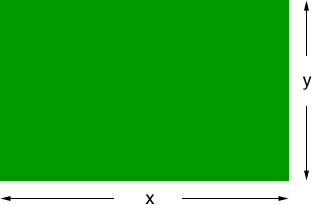Quandaries and Queries HI I AM LOUISE I AM DOING MY GCSE MATHS COURSEWORK ON FENCING A FARMER HAS FENCING OF 1000M AND WANTS A FIELD WITH THE BIGGEST POSSIBLE AREA HOW DO I GO ABOUT DOING THIS COURSEWORK YOU HAVE TO USE TRIGONOMENTRY AND PYTHAGORAS THEROEM Hi Louise, You didn't mention calculus so I am going to solve this for you without using calculus. I am going to assume that the field is a rectangle and has sides of length x meters and y meters.Since the farmer has 1000 m of fencing, 2x + 2y = 1000 and thus x + y = 500. If x and y are equal they are each 250 m and the field is a square. I want to think in terms of "How close is the field to a square?" Suppose that x = 250  + h then, since x + y = 500, y = 250 - h. Hence the area is (250 + h)(250 - h) = 2502 - h2 square meters In this form you can plainly see that 2502 - h2 is a maximum when h = 0, that is when the field is a square. Penny